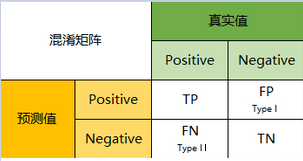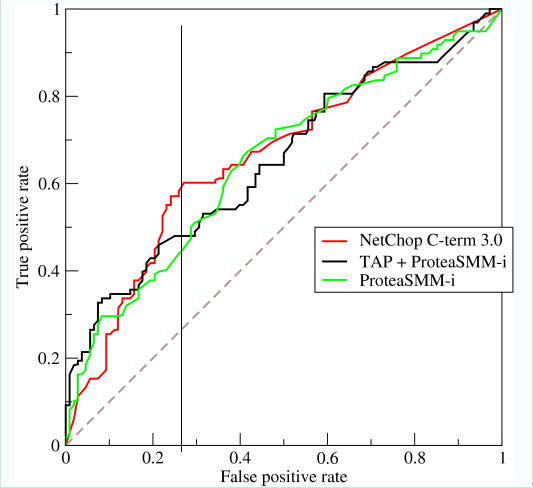# 评估指标 Metrics

## 混淆矩阵 Confusion Matrix## 二级指标

• 准确率(Accuracy) - 针对分类正确
• 精确率(Precision) - 所有阳性中分类正确的
• 灵敏度(Sensitivity) - 就是召回率(Recall), 也叫查全率.
• 特异度(Specificity)

## 三级指标

$$F_1 \ score = 2 \cdot \frac{Precision \cdot Recall} {Precision + Recall}$$

$$F_\beta \ score = (1+N^2) \cdot \frac{Precision \cdot Recall}{N^2\cdot Precision + Recall} = \frac{Precision \cdot Recall}{\frac{N^2}{1+N^2}\cdot Precision + \frac{1}{1+N^2}Recall}$$
$\beta$ 的取值范围是$[0, +\infty)$, 有以下特殊情况:

• ${\lim\limits_{N \to 0}}F_\beta \ score= Precision$
• $\beta = 1, F_\beta = F_1\ score$
• ${\lim\limits_{N \to +\infty}}F_\beta \ score= Recall$
• 能够从不同的$\beta$ 值看出, 如果$\beta$ 值越小, $Precision$ 越重要, 反之$\beta$ 值越大, $Recall$ 越重要.

## ROC曲线

ROC(Receiver Operating Characteristic) 受试者特性曲线. 纵坐标是true positive rate(TPR), 指的是所有实际为阳性的样本中被正确判定为阳性的比例. 横坐标是false positive rate(FPR), 指的是所有实际为阴性的样本中被错误判定为阳性的比例.
$$\displaylines{ TPR = \frac{TP}{TP+FN} \\ FPR = \frac{FP}{FP+TN} }$$上一篇机器学习之损失函数

2020-08-01循环神经网络小结
2020.09.07: 重写了LSTM和GRU的描述. 2020.08.22: 对部分内容进行了更新. 循环神经网络 Recurrent Neural Network面对时序型数据, 如自然语言, 乐谱, 金融数据等包含隐含的时间信息在
2020-07-30
目录